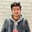Related Tags

computing

# What is a Turing machine?Danyal Ahmed

## Overview

In 1936, Alan Turing invented the Turing machine. He called it an automatic machine.

A Turing machine is a mathematical model. It accepts the recursively Enumerable languages, and consists of infinite length tape divided into cells on which input is given. The machine is more powerful than the PushDown Automata and Finite State Machine.

### Data structures

A tape sequence of infinite symbols contains a pointer of a tape head, and it points to the symbol on which the current control is present.

A tape head is either moving one step left or one step right depending upon the situation and having an option to read or write at that position.as

### How does the Turing machine work?

It consists of a tape head that reads from the input tape. Next, a state register stores the state of the Turing machine. The tape head reads from the input tape and is replaced according to the situation with another symbol. This changes its internal state. It then moves either to the left of the tape or the right. Finally, the input string is accepted if the Turing machine reaches the final state(F). Otherwise, it is rejected.

Here, it consists of 7 tuples:

• X: It defines a tape alphabet.
• ∑: It defines the input alphabet.
• Q: It defines the finite set of states.
• q0: It is necessary for the initial state.
• B: It is necessary for the blank symbol.
• F: It is necessary for the set of final states.
• δ: It is necessary for a transition function; here, the transition function is defined as δ: Q × X → Q × X × {Left_shift, Right_shift}.

### Example

Let's look at an example of a Turing machine {a^n*b^n where n>=1} given below:

### Explanation

• First of all, the input string is aaabbb in the input tape, and the head is at the first cell of the input tape.
• At state q0, it takes the a from the input tape head and replaces it with the symbol x, and then moves to the next state.
• At state q1, if it reads the a, it will be the same in that state; if it takes b, it replaces it with y to move to the next state.
• At state q2, it will repeat the same process as done in the previous two steps, but if it takes x from the head, then it will move to state q0.
• Now again, at state q0, if it takes y, it moves to the q3 state, and the rest of y will be accepted in state q3.
• At state q3, if it takes B, it moves to the final state (F), and the given string is accepted.

RELATED TAGS

computing

CONTRIBUTORDanyal Ahmed
RELATED COURSES

View all Courses

Keep Exploring

Learn in-demand tech skills in half the time